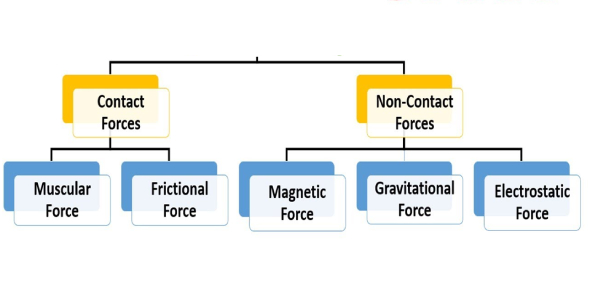# Types Of Forces MCQ Quiz: Exam!

9 Questions | Total Attempts: 543SettingsCreate your own Quiz.

• 1.
When a force pushes on an object to the right with a magnitude of 20N and another force pushes on the object to the left with a magnitude of 15N what would the resulting motion of the object be?
• A.

No motion

• B.

Toward the left

• C.

Toward the right

• D.

Upward

• 2.
In physical science, a push or a pull in a given direction is called a(n)...
• A.

Inertia

• B.

Accelerator

• C.

Force

• D.

Motion

• 3.
In a vector diagram, what does the length of the arrow represent?
• A.

The direction of the force

• B.

The magnitude or strength of the force

• C.

How long the force is

• D.

The area of the force

• 4.
Which of the following is an example of a non-contact force?
• A.

A child running through a field

• B.

A fan blowing a student's hair

• C.

A carpenter hammering a nail

• D.

A book falling off a desk

• 5.
The force that one surface exerts on another when the two rubs against each other are called...
• A.

Gravity

• B.

Acceleration

• C.

Inertia

• D.

Friction

• 6.
What does the head of each arrow indicate?
• A.

The magnitude of the force

• B.

The area of the force

• C.

The strength of the force

• D.

The direction of the force

• 7.
Which of the following is true of the buoyant force?
• A.

It makes an object feel heavier

• B.

It acts in the downward direction

• C.

It acts in the upward direction

• D.

It acts with the force of gravity

• 8.
The force that pulls a falling object toward the Earth is called...
• A.

Acceleration

• B.

Inertia

• C.

Momentum

• D.

Gravity

• 9.
Identify this piece of lab equipment.
• A.

Manual Balance

• B.

Spring Scale

• C.

Mechanical Scale

• D.

Triple Beam Scale

Related TopicsBack to top
×

Wait!
Here's an interesting quiz for you.